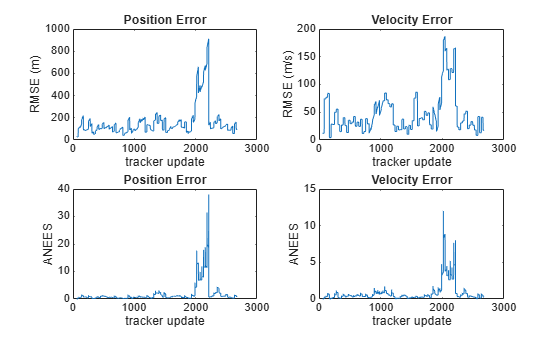# currentTruthMetrics

Metrics for recent truths

## Syntax

``metricsTable = currentTruthMetrics(errorMetrics)``

## Description

example

````metricsTable = currentTruthMetrics(errorMetrics)` returns a table of metrics, `metricsTable`, for every truth identifier provided in the most recent update.```

## Examples

collapse all

Examine the assignments and errors for a system tracking two targets.

First, load the stored track data.

`load trackmetricex tracklog truthlog`

Create objects to analyze assignment and error metrics.

```tam = trackAssignmentMetrics; tem = trackErrorMetrics;```

Create the output variables.

```posRMSE = zeros(numel(tracklog),1); velRMSE = zeros(numel(tracklog),1); posANEES = zeros(numel(tracklog),1); velANEES = zeros(numel(tracklog),1);```

Loop over all tracks to:

• Extract the tracks and ground truth at the i th tracker update.

• Analyze and retrieve the current track-to-truth assignment.

• Analyze instantaneous error metrics over all tracks and truths.

```for i=1:numel(tracklog) tracks = tracklog{i}; truths = truthlog{i}; [trackAM,truthAM] = tam(tracks, truths); [trackIDs,truthIDs] = currentAssignment(tam); [posRMSE(i),velRMSE(i),posANEES(i),velANEES(i)] = ... tem(tracks,trackIDs,truths,truthIDs); end```

Show the track metrics table.

`trackMetricsTable(tam)`
```ans=4×15 table TrackID AssignedTruthID Surviving TotalLength DeletionStatus DeletionLength DivergenceStatus DivergenceCount DivergenceLength RedundancyStatus RedundancyCount RedundancyLength FalseTrackStatus FalseTrackLength SwapCount _______ _______________ _________ ___________ ______________ ______________ ________________ _______________ ________________ ________________ _______________ ________________ ________________ ________________ _________ 1 NaN false 1120 false 0 false 3 3 false 0 0 false 0 0 2 NaN false 1736 false 0 false 8 88 false 0 0 false 28 3 6 3 true 1138 false 0 false 4 314 false 1 28 false 0 2 8 2 true 662 false 0 false 2 29 false 1 169 false 28 0 ```

Show the truth metrics table.

`truthMetricsTable(tam)`
```ans=2×10 table TruthID AssociatedTrackID DeletionStatus TotalLength BreakStatus BreakCount BreakLength InCoverageArea EstablishmentStatus EstablishmentLength _______ _________________ ______________ ___________ ___________ __________ ___________ ______________ ___________________ ___________________ 2 8 false 2678 false 4 168 true true 56 3 6 false 2678 false 3 645 true true 84 ```

Plot the RMSE and ANEES error metrics.

```subplot(2,2,1) plot(posRMSE) title('Position Error') xlabel('tracker update') ylabel('RMSE (m)') subplot(2,2,2) plot(velRMSE) title('Velocity Error') xlabel('tracker update') ylabel('RMSE (m/s)') subplot(2,2,3) plot(posANEES) title('Position Error') xlabel('tracker update') ylabel('ANEES') subplot(2,2,4) plot(velANEES) title('Velocity Error') xlabel('tracker update') ylabel('ANEES')```Show the current error metrics for each individual recorded track.

`currentTrackMetrics(tem)`
```ans=2×5 table TrackID posRMS velRMS posANEES velANEES _______ ______ ______ ________ ________ 6 44.712 20.988 0.05974 0.31325 8 129.26 12.739 1.6745 0.2453 ```

Show the current error metrics for each individual recorded truth object.

`currentTruthMetrics(tem)`
```ans=2×5 table TruthID posRMS velRMS posANEES velANEES _______ ______ ______ ________ ________ 2 129.26 12.739 1.6745 0.2453 3 44.712 20.988 0.05974 0.31325 ```

Show the cumulative error metrics for each individual recorded track.

`cumulativeTrackMetrics(tem)`
```ans=4×5 table TrackID posRMS velRMS posANEES velANEES _______ ______ ______ ________ ________ 1 117.69 43.951 0.58338 0.44127 2 129.7 42.8 0.81094 0.42509 6 371.35 87.083 4.5208 1.6952 8 130.45 53.914 1.0448 0.44813 ```

Show the cumulative error metrics for each individual recorded truth object.

`cumulativeTruthMetrics(tem)`
```ans=2×5 table TruthID posRMS velRMS posANEES velANEES _______ ______ ______ ________ ________ 2 258.21 65.078 2.2514 0.93359 3 134.41 48.253 0.96314 0.49183 ```

## Input Arguments

collapse all

Error metrics object, specified as a `trackErrorMetrics` System object™.

## Output Arguments

collapse all

Truth error metrics, returned as a table.

• When you set the `ErrorFunctionFormat` property of the input error metrics object to `'built-in'`, the table columns depend on the setting of the `MotionModel` property.

 Motion model Table Columns `'constvel'` `posRMSE`, `velRMSE`, `posANEES`, `velANEES`. `'constacc'` `posRMSE`, `velRMSE`, `accRMSE`, `posANEES`, `velANEES`, `accANEES` `'constturn'` `posRMSE`, `velRMSE`, `yawRateRMSE`, `posANEES`, `velANEES`, `yawRateANEES`

RMSE and ANEES denote root mean squared error and average normalized estimation error squared between a truth trajectory and its associated tracks at the current time step. Note that a truth trajectory can associate with multiple tracks. For example, the position RMSE and ANEES values for a truth are defined respectively as:

`$\begin{array}{l}posRMSE=\sqrt{\frac{1}{K}\sum _{k=1}^{K}‖\Delta {p}_{k}‖}\\ posANEES=\frac{1}{K}\Delta {p}_{k}^{T}{C}_{k}^{-1}\Delta {p}_{k}\end{array}$`

where K is the total number of tracks associated with the truth, Ck is the position covariance of the kth track at the current time step, and

`$\Delta {p}_{k}={p}_{track,k}-{p}_{truth}$`

is the position error between the kth associated track and the truth. The RMSE and ANEES values for other states (`vel`, `pos`, `acc`, and `yawRate`) are defined similarly.

• When you set the `ErrorFunctionFormat` property to `'custom'`, the table contains the arithmetically averaged values of the custom metrics output from the error function.

Introduced in R2018b